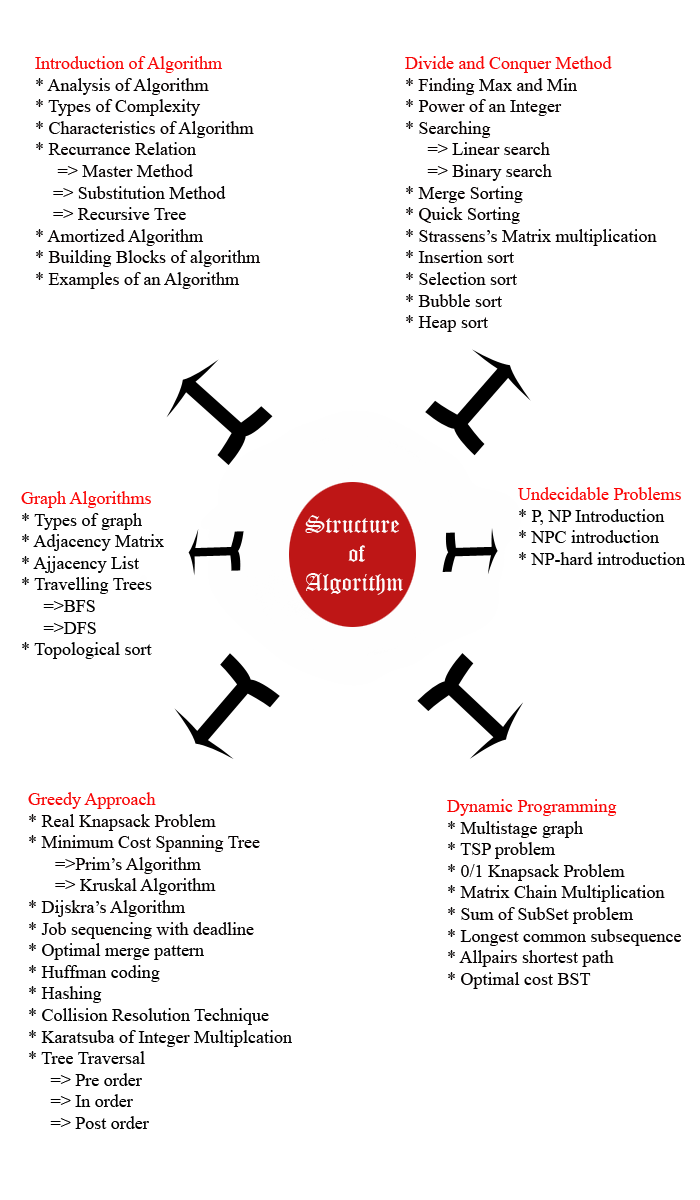## DESIGN AND ANALYSIS OF ALGORITHM

It's all about self learning.

Introduction of algorithm and Structure of algorithm
2 October, 2016

Algorithm: Its a combination of sequence of finite steps to solve a particular problem. The theoretical structure of computer program performance and resource usage. It helps to find out modularity, correctness, user-friendliness, simplicity, scalability, robustness, functionality and many more.

Let us consider one problm of preparing a Tea:
Now what is requirement we know already but how we will procede that will teach you what exactly algorithm does.
Step1: get a empty vessel
Step 2: get some water
a.    Do we have water??
1)    If yes, put it in the vessel.
2)    If no, do we want to buy water?
1.    If yes , then go out and buy.
2.    If no, we can terminate.
Step 3: Turn on the stove and move further..
Step 4 : Go ahead.
This is the most suitable problem to understand what is algorithm.
I can say you if you are a student of computer science, U have to deal with lot of problems regarding coding so first solve that problem with the help of algorithm and then move further to hard core section.
Algorithm will teach u the basics of programming and one thing I m telling u any problem in computers can't be solve without Algorithm and Theory of Computation. An algorithm is the step by step instructions to solve a given problem.

Why study algorithms and performance?
1.  Algorithms help us to understand scalability.
2.  Performance often draws the line between what is feasible and what is impossible.
3.  Algorithmic mathematics provides a language for talking about program behavior.
4.  The lessons of program performance generalize to other computing resources.

Here we will try to complete and learn all concept with theory and examples. Algorithm gives u the idea to solve a particular problem like if u r making a tea then what u need and how will u manage all the things is Algorithm.

### Goal of Analysis of algorithm:

The goal of analysis is to compare algorithms mainly in terms of running time but also in terms of other factors like memory , developers effoert etc.like as we know C complier is faster than Java compiler but java contains many things like Abstraction, Polymorphism.
Steps to construct Algorithm
Step1: Find out the problem
Step2: Find out the input and output (There is many inputs but output is only one).
Step3: Condition should be satisfied
Step4: Design the algorithm
Step5: Flow chart
Step6: Validation and testing
Step7: Implemenation
Step8: Analysis
Step9: Maintenance.
Step10: Output

Note: Step 4 and step 8 is main reason to call it Design and analysis of algorithm
Here is the structure of algorithm and course which we will learn in coming chapters:Final words of Notes one: Work with me , Enhance yourself , Learn yourself, Discover yourself..U will be on the top..so till den have fun and always visit my website..Thanks.